# ‘Impulse of Delight’: Paul Tod on MS17

In Trinity Term, the College invited me to write a short piece for the catalogue of the exhibition to mark the opening of the new Study Centre, describing two pages of the College’s manuscript MS17. This manuscript is a mathematical compilation, largely concerned with the methods needed for computing the date of Easter. The two pages chosen for display in the exhibition are evidently made up of tables of some kind but it takes a little effort to see exactly what kind. Having discovered what the two pages were about, I realised there would be no space to do justice to them in the confines of a catalogue note, but the pedagogic urge was too strong in me not to explain them, whence this longer note.

When you know what these pages are about, the age of MS17 is astonishing. It dates from about 1100 and the learning in it is from before the Norman Conquest, though the author seems to be a post-conquest monk with a Saxon name (Byrhtferth), and the composition is a tribute to the movement of scholars between Europe and England – long may it continue! The composition is roughly contemporary with Hildegard of Bingen, and with Abelard and Heloise. The mathematical content is in the service of computing the date of Easter, around which the religious year was organised, and which was a source of contention in the early church. For example the Celtic Church was essentially marginalised after the Synod of Whitby for its method of calculating Easter, which was held to be incorrect. The accepted definition of Easter Sunday was (and is) as the first Sunday after the first full moon after the spring equinox, with fine-tuning if any of these days coincided, and to compute it required knowledge of both solar and lunar calendars, the year-length and the interval between and dates of full moons. However, both equinoxes and full moons involved conventional`ecclesiastic’ definitions which with time drifted away from observational definitions of these astronomical events. The mathematical content of MS17 sits in an unbroken tradition back, in England at least, to Bede whose treatise on computing Easter is included elsewhere in MS17, but this tradition was not far from becoming obsolete. Astronomical calculations soon went over to base-60 methods (degrees, minutes, seconds) and became more accurate, and Arab learning arrived from Europe, bringing the astrolabe and the Ptolemaic system.

My aim here is to explain the tables on pages 48v and 49r in MS17, which are in fact multiplication and division tables and are held to be due to another monk, Hermannus of Reichenau. Reichenau is a monastery on an island in Lake Constance, and Hermannus was a remarkable figure. Also known as Hermannus Contractus or Herman the Lame, he was severely disabled from an early age, possibly from birth. Wikipedia asserts that he suffered from cerebral palsy and spina bifida but references a 1999 article by a German neurologist who has examined the evidence and concludes that motor neurone disease, possibly ALS, is a more likely diagnosis. Hermannus was placed in the monastery at the age of seven and became a complete polymath, eventually writing on mathematics, astronomy, history and musical theory, and also composing music, some of which you can find, with images of Reichenau, on Youtube.

To follow what I’m saying about the tables, you probably need to find images of them online. The whole manuscript can be found at: http://digital.library.mcgill.ca/ms-17/index.htm and you can download and zoom on the pages 48v and 49r.

We’ll start with 48v and let’s suppose we know that it is a multiplication table. (I should say that the arcade-like construction across the bottom of both pages is an abacus, on which one could multiple whole numbers by moving stones around). Down the left hand side of 48v we recognise the Roman numerals i to x, then xx increasing in tens to c, c increasing in hundreds to dcccc then what must be thousands written as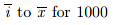to 10000.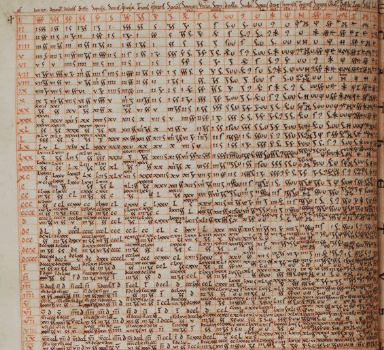The symbols at the top, however, are unfamiliar. There are twenty five columns and at the top of each is a symbol in red and above that a name in black.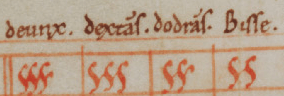Can we use the table to discover what the symbols mean? I’ll assume we can read the names, though in fact I’m getting them from the article of Florence Yeldham referenced at the end of this note. Here’s one way to decode the symbols, and I’ll describe another in the discussion of page 49r. Look first at the row labelled ii, so that is 2 (and is the first row, as there is no row for multiplication by one): in the 6th column there is an i or one, which arises from multiplying 2 by the fraction corresponding to this column, so this fraction must be ½ , and we now know its name, which is semis, and its symbol, which is like a Greek zeta  ζ ; now the 9th column meets the first row in the symbol for ½ , so this column corresponds to the fraction ¼, called quadrans and with a symbol like a zeta with a branch poking up (or a zeta proffering a tray). We can carry on like this – the 5th row, multiplication by 6, is particularly illuminating: in the second column there is a v or 5 so this column corresponds to 5/6; in the first column there’s a v followed by the symbol for ½ so that’s 5½ and so this column corresponds to 11/12 – and continuing like this we identify the first twelve columns as multiples of 1/12 from 11/12 down to 1/12, except that the 11th column is 1/8. Above the 12th column in the margin is a symbol like a division sign, which must be to help the medieval calculator keep track of which column they’re in, and as we’ll see below is also another symbol for 1/12 . Then the next thirteen columns are again a puzzle. For the first one past the mark, twice it is the symbol for 1/12 so it is 1/24 . Three times the next is 1/12 so its 1/36 ; four times the next is 1/12 so it’s 1/48 . Carrying on like this we find the following table of the fractions involved and their names. I’ve taken this from Yeldham. Note the top line has as for one, and this will be used when we get on to the next page, 49r. It has a symbol like a large plus sign and is visible in black at the top left of 48v and in red as in the last row in 49r.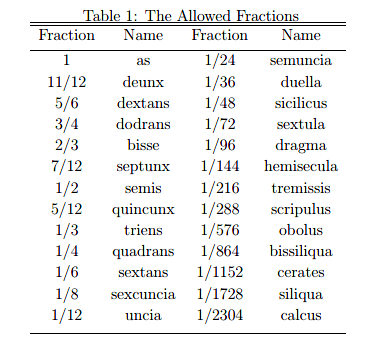Some of these names are a bit familiar – sextans, quadrans and triens are not a surprise – but the names in the second column look made up although the last, calcus, is reminiscent of calculus, meaning little stone which was used to describe operations (calculations) with an abacus before it was attached to terms like differential calculus.

Now this is very odd: only 25 fractions are considered or even admitted and they have individual names and distinct symbols. The second set are all unit fractions, in the sense that the numerator is one. The denominators are multiples of 12 up to 2304 which is 48 x 48, and 1/2304 is the smallest quantity admitted. For practical purposes this is perhaps not unreasonable – this fraction of a year is less than four hours. Let’s try some of the multiplications. From xL (find it on the left) times a duella (column 14) we expect to get 40/36=10/9=1+1/9, but 1/9 is not an allowed fraction. The solution adopted by the compiler of the table is to write it as a sum of allowed fractions. After a little playing around one notices that 1/9=1/12+1/36 or an uncia plus a duella, and zooming in on the relevant square of the table on 48v one sees it contains a Roman i and the symbols for an uncia and a duella, but no plus signs. So this is the convention: decompose the fractional part of the result into a sum of allowed fractions and juxtapose them, in order of size but without addition signs. There is a very long tradition in dealing only with unit fractions and decomposing the result of a calculation into a sum of unit fractions. The practice goes back at least to the Egyptians, and the Rhind papyrus in the British Museum, dating from about 1550 BC has tables of decompositions equivalent to sums likewhere a non-unit fraction (on the left) is decomposed as a sum of unit fractions. Returning to our tables, for a more ambitious calculation, go to the bottom row and column 19 – this is 10,000 times a tremisis or 10,000/216=46+8/27. Some more playing around gives 8/27=1/4+1/24+1/216, which is a quadrans plus a semuncia plus a tremisis. Zoom on this square to see xLvi for the 46 and then the three symbols for the fractional summands – Hermannus has it right!Now turn to page 49r: there are two tables, each triangular rather than square, and separated by a band labelled YPOTENUSA which is ‘diagonal’ in Latin and might best be read as ‘This space is intentionally blank’. Above the diagonal is a triangular table of the products of the allowed fractions with each other (it’s triangular because if you know a x b then you also know b x a and don’t need to write it again, so you only need to display cases with b greater than or equal to a). It’s easy to spot for example 1/2 x 1/2 = 1/4 – go across the top to the 6th column and then drop down to the diagonal. If the product is not an allowed fraction then as before it is decomposed as a sum of allowed fractions. For example one has in the second square down of the fourth column:

dextans x bissa = 5/6 x 2/3 = 5/9 = 1/2+1/24+1/72 = semis + semuncia + sextula.

It seems new rules could be invented as in (fifth square down column 19)

septunx x tremisis = 7/12 x 1/216

= 1/576 + 1/1728 + (2/3 x 1/1728) = obolus + silique + (bisse x silique),

which is correct but looks like cheating – you’ve expressed a product of allowed fractions only in terms of another product of allowed fractions. A larger problem looms up: there is a smallest allowed fraction 1/2304=1/48 x 1/48, which one can see where the 14th column meets the diagonal, but there are plenty of products of allowed fractions smaller than this – for example multiply 1/2×1/2304 then evidently this is less than the smallest fraction which has a name. What is to be done?

One way out is to invent a new notation. Look at the top right hand corner, row 1 column 25: this should be a deunx times a calcus or 11/12×1/2304 and is too small to have a name but in the square where we expect the answer we see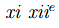before the sign for a calcus. This has to be read as eleven-twelfths: Hermannus has invented a new notation when he hits the limitations of the old one. You can see this again at a dextans times a calcus (the square below) written asof a calcus, or for a hemisecula times a hemisecula (where the 18th column meets the diagonal), which is 1/144×1/144 but the answer is given as aof a siliqua i.e. 1/12 of 1/1728 which is correct, and shows some calculational facility in the composer, but it’s not really an answer. There are some other oddities in this area over on the right of the table which must all arise from trying to handle fractions which aren’t admitted. There are frequent occurrences of  what look like abbreviations, dim and dimid, and of a symbol like a 7, for example in the 18th column. This 7 reappears in the lower table where we have an explanation for it but on this right-hand-side of the upper table it looks like one more ad hoc solution to a notational problem.

The table below the diagonal is a triangular table of quotients of theallowed fractions. It only displays quotients of an allowed fraction by a smaller allowed fraction (which is how it comes to be triangular: these are fractions a/b with less than or equal to a), so that the result is always greater than one, and consequently the order of the allowed fractions, down the left-hand border and down the diagonal, is reversed from what it has previously been: here it’s smallest first and largest last. Also the unit is admitted to the table: the bottom line is one (an as) divided by the allowed fractions, or equivalently it shows the reciprocals of the allowed fractions, and this gives confirmation of our calculation of the allowed fractions: one divided by a calcus is 2304 so a calcus is 1/2304, one divided by a siliqua is 1728 so a siliqua is 1/1728, and so on. This apparent facility with taking reciprocals may explain why the table is restricted to calculating a ÷ b  with a greater than b: if you want b ÷ a just take the reciprocal of a ÷ b.

The answers to these calculations are typically expressed as a Roman numeral for the whole-number part (which mathematicians prefer to call the integer part) followed by symbols for the allowed fractions. As an example, a deunx (penultimate row) divided by a sextans (16th column) is 11/12÷1/6=5½=v plus a semis, as shown. When the integer part is one, it is omitted in this part of the table, which might be a new convention but looks to me more like an error. You can see this phenomenon for example in the 19th column of the penultimate row, which should be 11/12÷1/2=1+5/6= plus a dextans; in the ‘answer’ square there’s a black dextans but also a red semis, whose purpose seems to be to keep track of which column you’re in, but the ‘one’ has been omitted (or forgotten).

Again a new problem is going to arise, for example from the bottom row and the 19th column, one divided by a quincunx should be 1÷5/12=12/5=2+2/5 but 2/5 is not an allowed fraction (and can’t be written as a sum of allowed fractions, as none of them has a factor 5 in the denominator) so the answer is given (in black) as 2 remainder 1/6 or 2 remainder a sextans and the remainder sign is like an Arabic numeral 7 (I found this interpretation in the article of Yeldham). Two squares to the right in this row we see one divided by a septunx equals 1÷7/12=1 remainder a quincunx, which is the answer given in black.

In the 14th column, which corresponds to an uncia or 1/12, every square but two contains what I called the division sign together with the answer given as a Roman numeral. The division sign seems to be to help the reader keep track of the column he or she is in so it’s functioning as another way to write an uncia.

I haven’t found many errors but there is one that’s easy to see: a septunx (6 rows up) divided by a calcus (1st column) is 7/12÷1/2304=1344 but the answer is given as īccc Lx iiii which is 1364 – the Lx should be xL.

It’s worth saying that a multiplication table for fractions could be useful, so that 49r is not just ornamental if you want to multiply 365¼ by 18¾ choosing two numbers which look vaguely astronomical, you could split the calculation into four parts:

365 × 18, 365 × 3/4, 18 ×1/4, and 3/4 × 1/4,

and add the four results. Here the first part is found with an abacus, the second and third by the table on 48v and the last by the table above the diagonal on 49r.

A division table for fractions, like that below the diagonal on 49r, could just about be used if one knew about reciprocals and it seems from the bottom line of the table below the diagonal that Hermannus probably did. Thus if you want to divide N1+F1 by N2+F2, where N1,N2 are integers and F1,F2 are fractions less than one then the quotient can be expanded as

(N1+F1)/(N2+F2) =1/(N2/N1+F2/N1)+1/(N2/F1+F2/F1).

This is anachronistic in as much as the algebra I used for this wasn’t available in 1100, but they were ingenious and could have found another way to the answer. This calculation requires four steps to start: calculate N2/N1 by abacus (it is possible to divide integers with an abacus) and then N1/F2, N2/F1 and F2/F1 by 49r, possibly doing a couple of reciprocals. Then three more reciprocals need calculating. I don’t say it was done this way but it could have been. Alternatively the second table on 49r could just be showing off!

I want to finish with three anecdotes about the persistence of the numbers used in these calculations, and the persistence of the impulses behind the calculations and the manuscript. The first connects to the naming of individual numbers, as for example a dextans for 5/6 or a septunx for 7/12. I want to say: if they have names then you know them in a different way. It was famously said by the mathematician Hardy of the mathematician Ramanujan that ‘the integers were his personal friends’ and illustrated by a story of Hardy visiting Ramanujan in Putney where he was lying unwell. ‘My taxi here had a most uninteresting number’ said Hardy, ever the razor-sharp conversationalist, ‘1729’. ‘On the contrary’ responded Ramanujan ‘That is the smallest number expressible as a sum of two cubes in two different ways’, which is the observation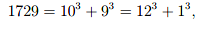together with the claim that this can’t be done for a positive number smaller than 1729.

For the second, as a child I was a passionate maker of Airfix plastic construction kits of aircraft, which were all to a scale 1/72 of life size, or a sextula as we now know to say. I checked this on Amazon recently to find that Airfix have branched out and their aircraft kits are now also made in scales 1/24 (a semuncia), 1/48 (a sicilicus), and 1/144 (a hemisecula). This pattern isn’t followed with cars (1/32), tanks (1/76), or boats (1/600), so why in aircraft?Finally the beautiful decoration of MS17 can’t fail to catch the eye, even on these pages of tables where four colours are used and the abacus arcade at the bottom is ‘coloured in’. Aged about 8, I had a teacher in primary school, Mr Plummer, who according to legend had come out of retirement to teach us and who was passionate for water colours. After instructing us in constructions in Euclidean geometry, such as the circumcircle of a given triangle or even the in-circle of a triangle, Mr Plummer had us take out our brushes and colour in the separate regions of the diagram, evidently feeling, and instilling in us, the same impulse of delight felt by the 12th century composer of MS17.

Paul Tod. 8/11/2019.

In writing this note, I’ve drawn heavily on Fraction Tables of Hermannus Contractus by Florence A. Yeldham in Speculum Vol. 3. No. 2 (Apr.1928), pp. 240-245, available on JSTOR.

This post was written by Emeritus Research Fellow,  Prof. Paul Tod, to celebrate the Michaelmas Term 2019 exhibition, Special Collections Today and Tomorrow, which marked the opening of the new Library & Study Centre. The exhibition is available to visit until 13th December 2019. MS17 is located in a display case by the Old Library.